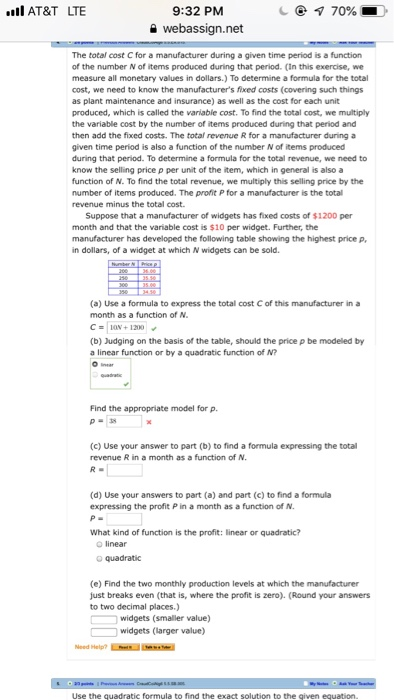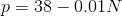# i dont have anyore info to provdie AT&T LTE е 70% 9:32 PM 을 webassign.net The total cost C for a manufacturer during a given time period is a function of the number N of items produced duri...i dont have anyore info to provdie
AT&T LTE е 70% 9:32 PM 을 webassign.net The total cost C for a manufacturer during a given time period is a function of the number N of items produced during that period. (In this exercise, we measure all monetary values in dollars.) To determine a formula for the total cost, we need to know the manufacturer's fixed costs (covering such things as plant maintenance and insurance) as well as the cost for each unit produced, which is called the variable cost. To find the total cost, we multiply the variable cost by the number of items produced during that period and then add the fixed costs. The total revenue R for a manufacturer during a given time period is also a function of the number N of items produced during that period. To determine a formula for the total revenue, we need to know the selling price p per unit of the item, which in general is also a function of N. To find the total revenue, we multiply this selling price by the number of items produced. The profit P for a manufacturer is the total revenue minus the total cost Suppose that a manufacturer of widgets has fixed costs of $1200 per month and that the variable cost is$10 per widget. Further, the manufacturer has developed the following table showing the highest price p in dollars, of a widget at which N widgets can be sold. (a) Use a formula to express the total cost C of this manufacturer in a month as a function of N. CN+1200 (b) Judging on the basis of the table, should the price p be modeled by a linear function or by a quadratic function of N Find the appropriate model for p. (c) Use your answer to part (b) to find a formula expressing the total revenue R in a month as a function of N. (d) Use your answers to part (a) and part (c) to find a formula expressing the profit P in a month as a function of N What kind of function is the profit: linear or quadratic? O linear O quadratic (e) Find the two monthly production levels at which the manufacturer just breaks even (that is, where the profit is zero). (Round your answers to two decimal places.) widgets (smaller value) widgets (larger value) Use the quadratic formula to find the exact solution to the qiven equation.

(a)(b) The price p must be modeled by a linear equation since the decrease in the value of P is constant with the increase in 50 units of N

The points are (200,36) and (250,35.50)

Slope = (35.50-36)/(250-200) = -0.50/50 = -0.01

Using the slope point form, we can write the equation asHence the price function is given by p = 38 - 0.01N

(c)(d) The profit function can be determined by subtracting the cost function from the revenue functionSince the profit contains N^2 term, hence it is a quadratic function

(e)

For break even, the profit must be equal to zeroSolving the quadratic equation we get

N = 43.53 (smaller value)

N = 2756.47 (larger value)

Note - Post any doubts/queries in comments section.

##### Add Answer of: i dont have anyore info to provdie AT&T LTE е 70% 9:32 PM 을 webassign.net The total cost C for a manufacturer during a given time period is a function of the number N of items produced duri...
More Homework Help Questions Additional questions in this topic.